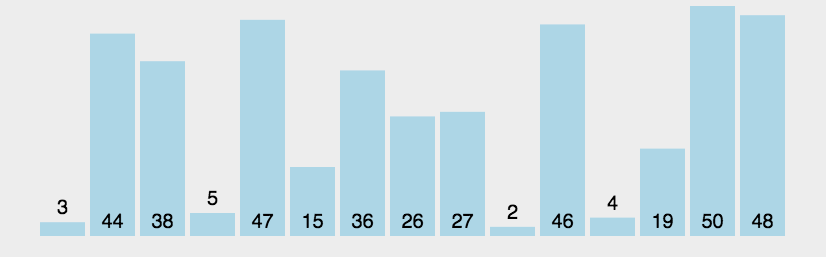十大经典排序算法之冒泡排序

【冒泡排序】（BubbleSort）a)原理冒泡排序是最简单的排序算法。它依次比较相邻的两个元素，如果它们的顺序错误就把它们交换过来，它重复的查询所要排序的数列。查询数列的工作直到不需要再进行交换元素即停止。排序过程中越小的元素经过交换依次冒泡浮岛数列的始端，由此得名冒泡。b)演示动态图c)算法步骤l比较相邻元素。如果前者比后者大，则进行交换；l依次查询数列中相邻元素，并进行比较，因此，一轮查询后...【冒泡排序】（Bubble Sort

a) 原理

b) 演示动态图c) 算法步骤

l 比较相邻元素。如果前者比后者大，则进行交换；

l 依次查询数列中相邻元素，并进行比较，因此，一轮查询后，最大元素位于末端；

l 针对剩余数列元素重复上述查询比较工作；

l 重复上述步骤，直到数列排序完成。

d) 代码实现

#include <iostream>using namespace std;//函数声明void BubbleSort(int[],int);//冒泡排序void ShowArray(int[],int);//打印//主函数int main(){ int array_a[] = {5,6,8,2,4,62,31,58,94,50,16}; int len = sizeof(array_a)/sizeof(array_a); //打印数组 cout << "原始数组： "; ShowArray(array_a,len); //1-冒泡排序 BubbleSort(array_a,len); //打印数组 cout << "【冒泡】排序后数组： "; ShowArray(array_a,len); return 0;}//函数实现之打印数组void ShowArray(int array_a[],int len){ for(int i=0;i<len;i  ) {  cout << array_a[i] << "  "; } cout << endl;}//函数实现之冒泡排序：分为外层和内层；void BubbleSort(int array_a[],int len){ for (int i=0;i<len-1;i  ) //外层控制比较的轮数； {  for (int j=0;j<len-i-1;j  )//内层控制每轮比较的次数；  {if (array_a[j]>array_a[j 1]){ int temp; temp = array_a[j]; array_a[j] = array_a[j 1]; array_a[j 1] = temp;}  } }}